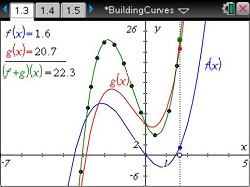# Activities

••• ##### Subject Area

• Math: Algebra II: Polynomials

• ##### Author9-12

45 Minutes

• ##### Device
•TI-Nspire™ CX/CX II
•TI-Nspire™ CX CAS/CX II CAS
• TI-Nspire™
• TI-Nspire™ CAS
• ##### Software

TI-Nspire™
TI-Nspire™ CAS

3.0

## Building Curves

#### Activity Overview

Students approach performing the basic operations on the polynomials from a graphical perspective. Given the graphs of two functions, they plot points that lie on the graph of the sum of the functions and draw conclusions about its behavior. Next, they calculate a regression to fit the points they plotted. Finally, they find the sum of the functions algebraically and compare it with the result of the regression.

#### Key Steps

•In this problem, students build the graph of (f + g)(x) from the graphs of f(x) and g(x). Before moving past the introduction on page 1.2, discuss what (f + g)(x) means and make hypotheses about what the graph of (f + g)(x) will look like. Ask: If f(x) = 2 and g(x) = 3, what will (f + g)(x) look like? Students should find that, for the graph of (f + g)(x), each y‑value is simply the sum of f(x) and g(x). Page 1.4 shows the graphs of f(x) and g(x).

Students move the slider on the x‑axis to choose an x-value, and the values of f(x) and g(x) update. The other point on the dotted line can be moved to set the y or f(x) value. Students will use this point to mark points on the curve (f + g)(x).